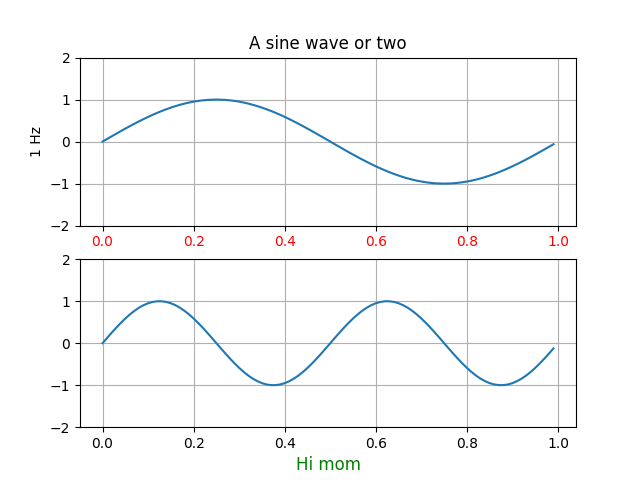Pythonic Matplotlib¶

Some people prefer to write more pythonic, object-oriented code rather than use the pyplot interface to matplotlib. This example shows you how.

Unless you are an application developer, I recommend using part of the pyplot interface, particularly the figure, close, subplot, axes, and show commands. These hide a lot of complexity from you that you don’t need to see in normal figure creation, like instantiating DPI instances, managing the bounding boxes of the figure elements, creating and realizing GUI windows and embedding figures in them.

If you are an application developer and want to embed matplotlib in your application, follow the lead of examples/embedding_in_wx.py, examples/embedding_in_gtk.py or examples/embedding_in_tk.py. In this case you will want to control the creation of all your figures, embedding them in application windows, etc.

If you are a web application developer, you may want to use the example in webapp_demo.py, which shows how to use the backend agg figure canvas directly, with none of the globals (current figure, current axes) that are present in the pyplot interface. Note that there is no reason why the pyplot interface won’t work for web application developers, however.

If you see an example in the examples dir written in pyplot interface, and you want to emulate that using the true python method calls, there is an easy mapping. Many of those examples use ‘set’ to control figure properties. Here’s how to map those commands onto instance methods

The syntax of set is:

plt.setp(object or sequence, somestring, attribute)

if called with an object, set calls:

object.set_somestring(attribute)

if called with a sequence, set does:

for object in sequence:
object.set_somestring(attribute)

So for your example, if a is your axes object, you can do:

a.set_xticklabels([])
a.set_yticklabels([])
a.set_xticks([])
a.set_yticks([])import matplotlib.pyplot as plt
import numpy as np

t = np.arange(0.0, 1.0, 0.01)

fig, (ax1, ax2) = plt.subplots(2)

ax1.plot(t, np.sin(2*np.pi * t))
ax1.grid(True)
ax1.set_ylim((-2, 2))
ax1.set_ylabel('1 Hz')
ax1.set_title('A sine wave or two')

ax1.xaxis.set_tick_params(labelcolor='r')

ax2.plot(t, np.sin(2 * 2*np.pi * t))
ax2.grid(True)
ax2.set_ylim((-2, 2))
l = ax2.set_xlabel('Hi mom')
l.set_color('g')
l.set_fontsize('large')

plt.show()

Keywords: matplotlib code example, codex, python plot, pyplot Gallery generated by Sphinx-Gallery